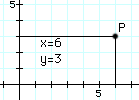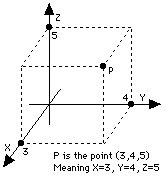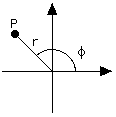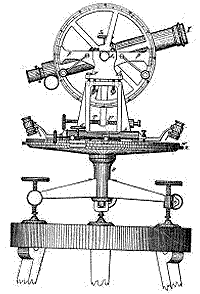# (5c) CoordinatesIndex5.Latitude and
Longitude5a. Navigation5b. Cross-Staff5c. Coordinates6. The Calendar6a. Jewish Calendar7.Precession8. The Round Earth8a. The Horizon8b. Parallax
Coordinates are sets of numbers that describe position-- position along a line, on a surface or in space. Latitude and longitude, or declination and right ascension, each is a system of coordinates on the surface of a sphere--on the globe of the Earth or the globe of the heavens.

## Coordinates on a Flat PlaneRené Descartes

A more widely used system are cartesian coordinates, based on a set of axes perpendicular to each other. They are named for Rene Descartes ("Day-cart"), a French scientist and philosopher who back in the 1600s devised a systematic way of labeling each point on a flat plane by a pair of numbers. You may well be already familiar with it.

The system is based on two straight lines ("axes"), perpendicular to each other, each of them marked with the distances from the point where they meet ("origin") as in the drawing below. The axes continue to the left of the origin and below it, and are marked there with negative numbers.The distance on one axis is named "x" and on the other axis "y". Given then a point P, one draws from it lines parallel to the axes, and the values of x and y at their intersections completely define the point. In honor of Descartes, this way of labeling points is known as a cartesian system and the two numbers (x,y) that define the position of any point are its cartesian coordinates.

Graphs use this system, as do some maps.This works well on a flat sheet of paper, but the real world is 3-dimensional and sometimes it is necessary to label points in 3-dimensional space. The cartesian (x,y) labeling can be extended to 3 dimensions by adding a third coordinate z. If (x,y) is a point on the sheet, then the point (x,y,z) in space is reached by moving to (x,y) and then rising a distance z above the paper (points below it have negative z).

Very simple and clear, once a decision is made on which side of the sheet z is positive. By common agreement the positive branches of the (x,y, z) axes, in that order, follow the thumb and the first two fingers of the right hand when extended in a way that they make the largest angles with each other.

What follows uses the trigonometric functions sine and cosine; if these are not familiar to you, either skip the rest of the section, or go learn about them.

## Polar CoordinatesCartesian c oordinates (x,y) are not the only way of labeling a point P on a flat plane by a pair of numbers. Other ways exist, and they can be more useful in special situations.

One system ("polar coordinates") uses the length r of the line OP from the origin to P (i. e. the distance from P to the origin) and the angle that line makes with the x-axis. Angles are often denoted by Greek letters, and here we follow conventions by marking it with φ (Greek f). Note that while in the cartesian system x and y play very similar roles, here roles are divided: r gives distance and φ direction.

The two representations are closely related. From the definitions of the sine and cosine

x = r cos φ
y = r sin φ

r2 = x2 + y2

Once r is known, the rest is easy

cos φ = x/r
sin φ = y/r

These relations fail only at the origin, where x = y = r = 0. At that point, φis undefined and one can choose for it whatever one pleases.

In three dimensional space, the cartesian labeling (x,y,z) is nicely symmetric, but sometimes it is convenient to follow the style of polar coordinates and label distance and direction separately. Distance is easy: you take the line OP from the origin to the point and measure its length r. You can even show from the theorem of Pythagoras that in this case

r2 = x2 + y2 + z2

All the points with the same value of r form a sphere of radius r around the origin O. On a sphere we can label each point by latitude λ (lambda, small Greek L) and longitude φ (phi, small Greek F), so that the position of any point in space could defined by the 3 numbers (r, λ, φ).

## Azimuth and ElevationAn old surveyor's tele-  scope (theodolite).

The surveyor's telescope is designed to measure two such angles. The angle φ is measured counterclockwise in a horizontal plane, but surveyors (and soldiers) work with azimuth, a similar angle measured clockwise from north. Thus the directions (north, east, south, west) have azimuth (0°, 90°, 180°, 270°). A rotating table allows the telescope to be pointed in any azimuth.

The angle λ is called elevation and is the angle by which the telescope is lifted above the horizontal (if it looks down, λ is negative). The two angles together can in principle specify any direction: φ ranges from 0 to 360°, and λ from –90° (straight down or "nadir") to +90° (straight up or "zenith").

Again, one needs to decide from what direction is the azimuth measured--that is, where is azimuth zero? The rotation of the heavens (and the fact most humanity lives north of the equator) suggests (for surveyor-type measurements) the northward direction, and this is indeed the usual zero point. The azimuth angle (viewed from the north) is measured counterclockwise.

Mathematicians however prefer their own notation and replace "latitude" (or elevation) λ with co-latitude θ= 90 – λ deg., the angle not to the horizon but to the vertical. The angle θ (theta, one of two t-s in Greek) goes from 0 to 180°, not from –90° to + 90°. This actually may make more sense, because it is easier to measure an angle between two lines (OP and the vertical) rather than between a line and a flat plane (OP and the horizontal).]

[And in case you have to know: In 3 dimensions, when deriving the spherical coordinates (r, θ, φ) that correspond to cartesian (x,y,z) from the same origin, θ is measured from the +z-axis and φ is measured in the (x,y) plane, counterclockwise from the x-axis as seen from the +z side.   This needs to be said, because viewed from –z ("from below") clockwise becomes counterclockwise, and vice versa.]

Questions from Users:
***     Drawing a Perpendicular Line in Rectangular Coordinates

Next Stop: #6 The Calendar

Author and Curator:   Dr. David P. Stern
Mail to Dr.Stern:   stargaze("at" symbol)phy6.org .

Last updated: 10 October 2016